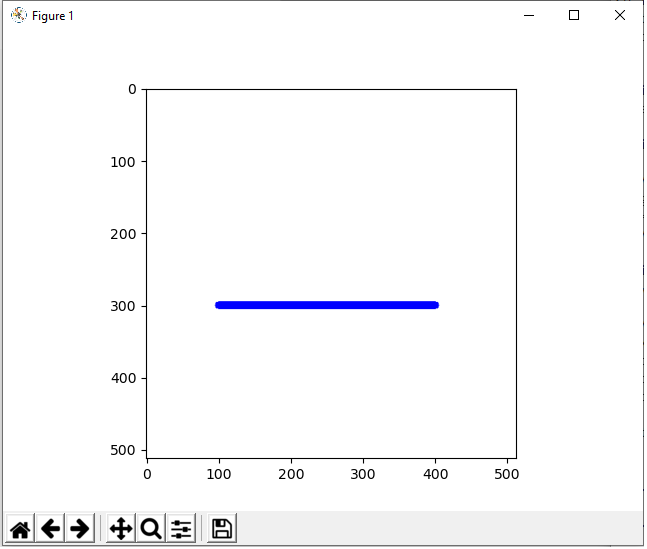﻿ How to Draw a Line in Python using OpenCV ﻿# How to Draw a Line in Python using OpenCVIn this article, we show how to draw a line in Python using the OpenCV module.

OpenCV allows a user to create a wide variety of shapes, including rectangles, squares, lines, etc.

Python has a built-in line() function, which allows us to add a line to an image, usually a blank one. We create this blank image with numpy. Then using OpenCV, we add our line to it.

There are many parameters that go into the line() function, which allow us to control various aspects of it.

This includes its location (where it is on the blank image such as to the left, to the right, middle, upper, lower, etc.). It also includes its length, color, and border thickness.

For our first example, we're going to build a simple horizontal line.

The line we will create is shown below.The code to create this line which is shown above is shown below.

Let's now go over this code.

First, we import all the modules we need which is cv2 (OpenCV), numpy (to create a blank image), and matplotlib (to get grided axes).

Next, we create a blank white image and store this in the variable, whiteblankimage. This is done using numpy.

We now have a blank image (like a canvass) in which we can now draw our line.

Next, we use the cv2.line() function to create our line on this white blank image.

The first parameter that we must feed into the cv2.line() function is the image we want to draw it on. We will be using what we created with numpy, whiteblankimage. This provides a perfect clean white background, in which we can place our line shape.

The second parameter we must specify is where the line starts. This is specified through the pt1 attribute (standing for point 1). We want our line, in this example, to begin at the point, (100,300). If you're not sure where to begin, show the blank image with grids first before beginning the line. Then you can see the dimensions of where you want this line to be placed.

The third parameter we must specify is where the line ends. This is specified through the pt2 attribute (standing for point 2). In this example, we want our line to end at (400,300). This makes for a horizontal length of 300.

Next, in the fourth parameter, we must specify the color of the line. We specify the color with the RGB format. Since in this example our line is blue, this is represented by (0,0,255).

In the last parameter, the fifth parameter, we specify the thickness of the border of the line. In this case, it's 10, which is relatively thick for a border.

So, in the above example, we created a simple horizontal line. Of course, the line doesn't have to be horizontal. It can also be vertical or it can be diagonal. We create vertical and diagonal lines by changing the starting and ending points of the line.

Shown below is a vertical line.The code to create this vertical line is shown below.

Next we create a diagonal line.

This again is created by the specifying the start and end points of the line.

A diagonal line we created is shown below.The code to create this diagonal line is shown below.

Lastly, you can place however many lines (and other shapes) you want on an image by specifying multiple lines.

In the image below, we have 2 diagonal lines forming an X.The code to create these 2 diagonal lines forming an X is shown below.

So you can do multiple things with lines in Python and even use them to create symbols or other shapes.

And this is how to draw a line in Python using the OpenCV module.

Related Resources